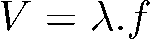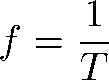Sound and Oscillation

# mechanical waves

Mechanical waves are vibrations or disturbances in the medium carrying energy. These waves do not carry matter and need a medium to propagate, unlike electromagnetic waves.

The classification of mechanical waves is based on the direction of propagation and vibration. As for propagation, they can be: transverse or longitudinal. As for vibration, they can be: one-dimensional, two-dimensional and three-dimensional.

Like any wave, mechanics have: amplitude (A), frequency (f), wavelength (λ) and velocity (v). To find the velocity, just use the equation: v= λ.f.

## Summary

• Mechanical wave is a vibration of the medium.
• Mechanical waves transport energy but not matter.
• We classify waves with respect to the direction of vibration and propagation.
• The characteristics of the wave are: amplitude (A), frequency (f), wavelength (λ) and velocity (v).
• To calculate the wave speed, just use the equation: v = λ.f.
• To calculate the frequency of the wave, just use the equation: f=1/T.

## What are mechanical waves?

Waves are disturbances that propagate carrying energy. In the case of mechanical waves, they need a medium to propagate and, like any wave, cannot carry matter.

## Main features of mechanical waves

The main characteristics of mechanical waves are related to their nature and shape.

• Mechanical waves do not transport matter, only energy.
• They need a medium to propagate, such as air.
• They interact with the environment around them by vibrating the molecules.
• They undergo phenomena such as: refraction (wave changes medium), reflection (wave encounters an obstacle and returns), diffraction (wave goes around obstacles) and interference (wave encounters another wave).
• They have amplitude (wave strength), velocity (wave speed), frequency (wave oscillation) and wavelength (wave size):

→ Amplitude (A ): is the height that the wave can reach. The high points of the wave (crests) are where we find the maximum amplitude and the low points (valleys) are known as the minimum amplitude. Its unit of measurement is meters (m).

→ Velocity of propagation (V) : it is the velocity of the wave in the medium. Its unit of measurement is m/s.

→ Frequency (f) : it is the complete oscillation of a wave. Its unit of measurement is the hertz (Hz).

→ Wavelength (λ) : is the space that the wave travels to complete one oscillation (one cycle). The lambda (λ) is found between two crests (highest point of the wave) or two valleys (lowest point of the wave). Its unit of measurement is meters (m).

## Types of mechanical waves

Mechanical waves can be classified in two ways: in relation to their direction of vibration and their direction of propagation.

With respect to the direction of vibration, a wave can be:

• Transversal : the vibration made to produce the wave is perpendicular (forms a 90° angle) to its propagation. Example of a transverse wave: a stone falling into a puddle of water.
• Longitudinal : the vibration that produces the wave is parallel (same direction) to its propagation. Longitudinal wave example: sound waves .

Regarding its propagation direction, one can be:

• One- dimensional : propagates in only one direction. Example: a guitar string.
• Two- dimensional : propagates in up to two directions. Example: a stone falling into a lake.
• Three- dimensional : It propagates in several directions. Example: a sound wave.

## How to calculate the speed of mechanical waves?

To find how fast the wave propagates (velocity), we need the size of its wavelength (λ) and its frequency (f):• V = wave speed (m/s)
• λ = wavelength (m)
• f = frequency (Hz)

The frequency of the wave can be found through the period of the wave (T), which is the time to complete a wave:## Difference between mechanical waves and electromagnetic waves

The main difference between mechanical and electromagnetic waves is related to their propagation. While mechanical waves need a medium to propagate, electromagnetic waves do not need a medium and can propagate even in a vacuum (where there is no matter).

Electromagnetic waves have a spectrum divided into seven types of waves: radio, microwave, infrared, visible light, ultraviolet, x-rays and gamma rays. From left to right, we have an increase in wave frequency.

## Solved exercises on mechanical waves

1. (Enem 2012) On a day of very heavy rain, a leak was observed over the center of an indoor pool, forming a pattern of circular waves. In this situation, it was observed that two drops fell every second. The distance between two consecutive crests was 25 cm and each one approached the edge of the pool with a speed of 1.0 m/s. After some time the rain stopped and the drip started to fall once a second.

With the decrease in rainfall, the distance between the crests and the propagation speed of the wave became, respectively,

a) greater than 25 cm and greater than 1.0 m/s.

b) greater than 25 cm and equal to 1.0 m/s.

c) less than 25 cm and less than 1.0 m/s.

d) less than 25 cm and equal to 1.0 m/s.

e) equal to 25 cm and equal to 1.0 m/s.

Resolution

Alternative B.

In the first situation, we have two drops falling per second. This can be described as wave frequency, and the distance between two crests is what we call the wavelength, that is, in the first situation, with heavy rain, we have f = 2 Hz, v = 1 m/s and λ = 25 cm or 0.25 m.

The wave speed only changes if the medium changes. As this does not occur in the exercise, we know that the final velocity will remain the same.

With the wave velocity equation, we can see the relationship between frequency and wavelength:If we isolate the frequency, we realize that it is inversely proportional to the wavelength, that is, if one increases, the other decreases and vice versa.So as the rainfall decreases, the frequency also decreases, and so we know that the wavelength has increased.

2.  (Unesp 2013) The image obtained in a didactic laboratory represents circular waves produced on the surface of the water in a wave tank and, highlighted, three crests of these waves. The center generating the waves is point P, periodically disturbed by a vibrating rod.

Considering the information in the figure and knowing that the speed of propagation of these waves on the surface of the water is 13.5 cm/s, it is correct to state that the number of times that the rod touches the surface of the water, each second, is equal to :

a) 4.5.

b) 3.0.

c) 1.5.

d) 9.0.

e) 13.5.

Resolution

alternative D.

From the statement, we have that the wave speed is 13.5 cm/s and, from the image, we see that, in three crests, we have a distance of 3 cm, but the wavelength (λ) is measured between two crests, or that is, from the image, we have that λ=1.5 cm.

Isolating the frequency in the velocity equation and putting the information in the utterance, we have: